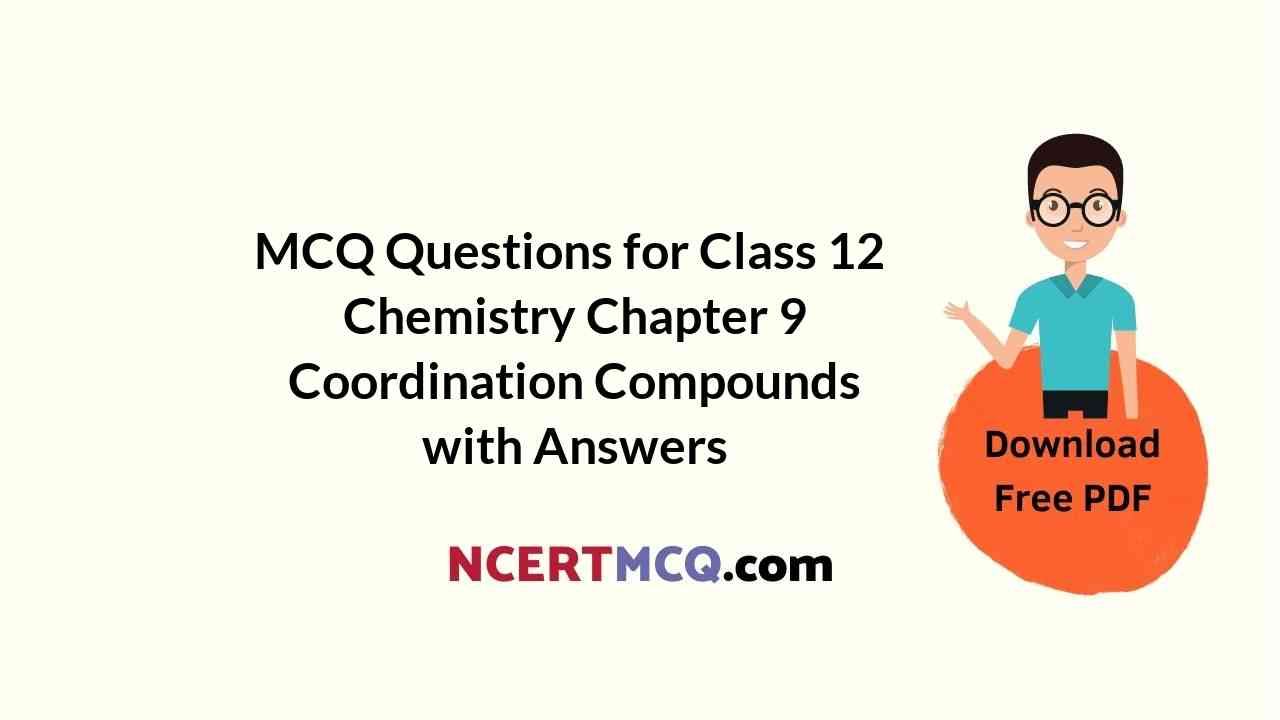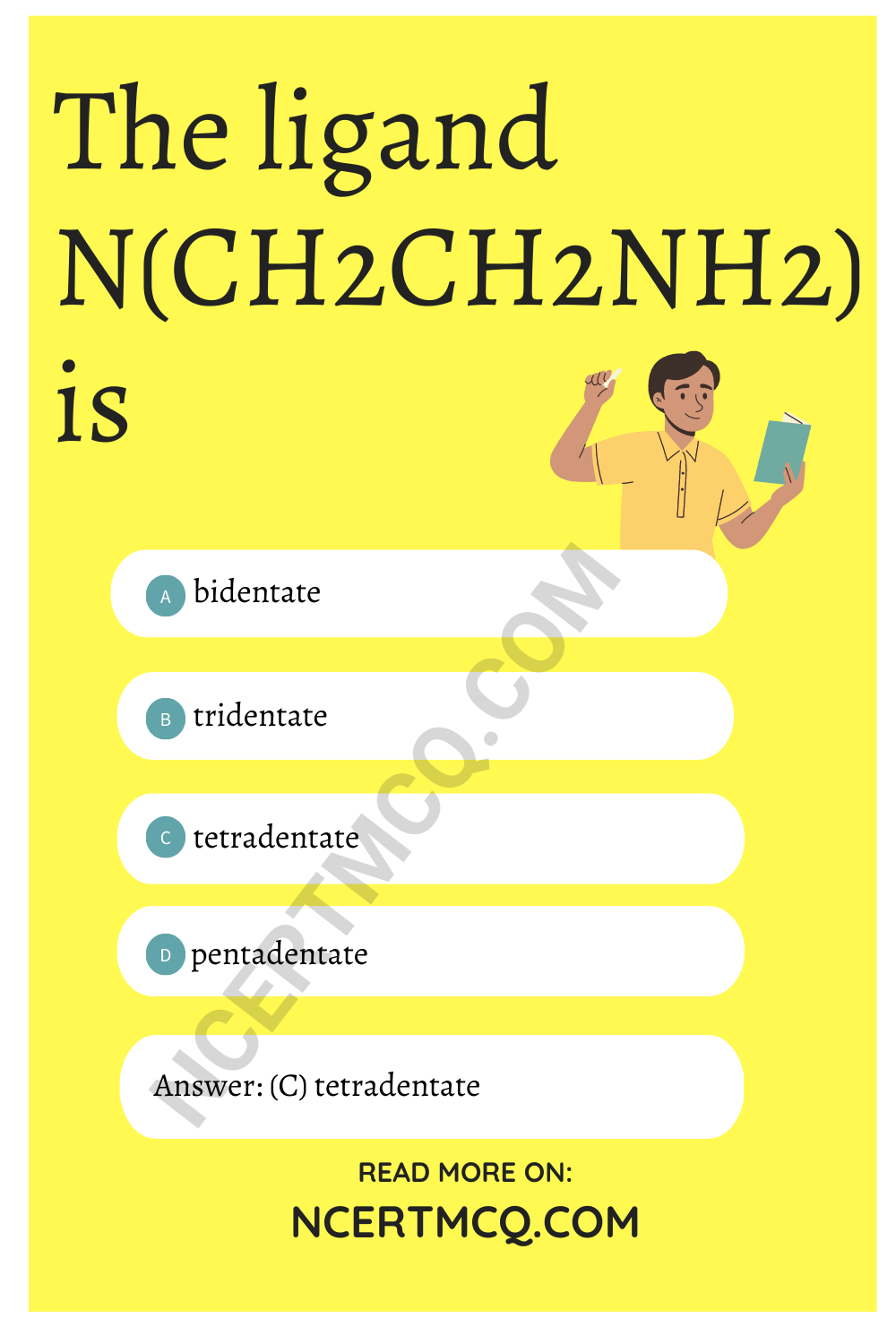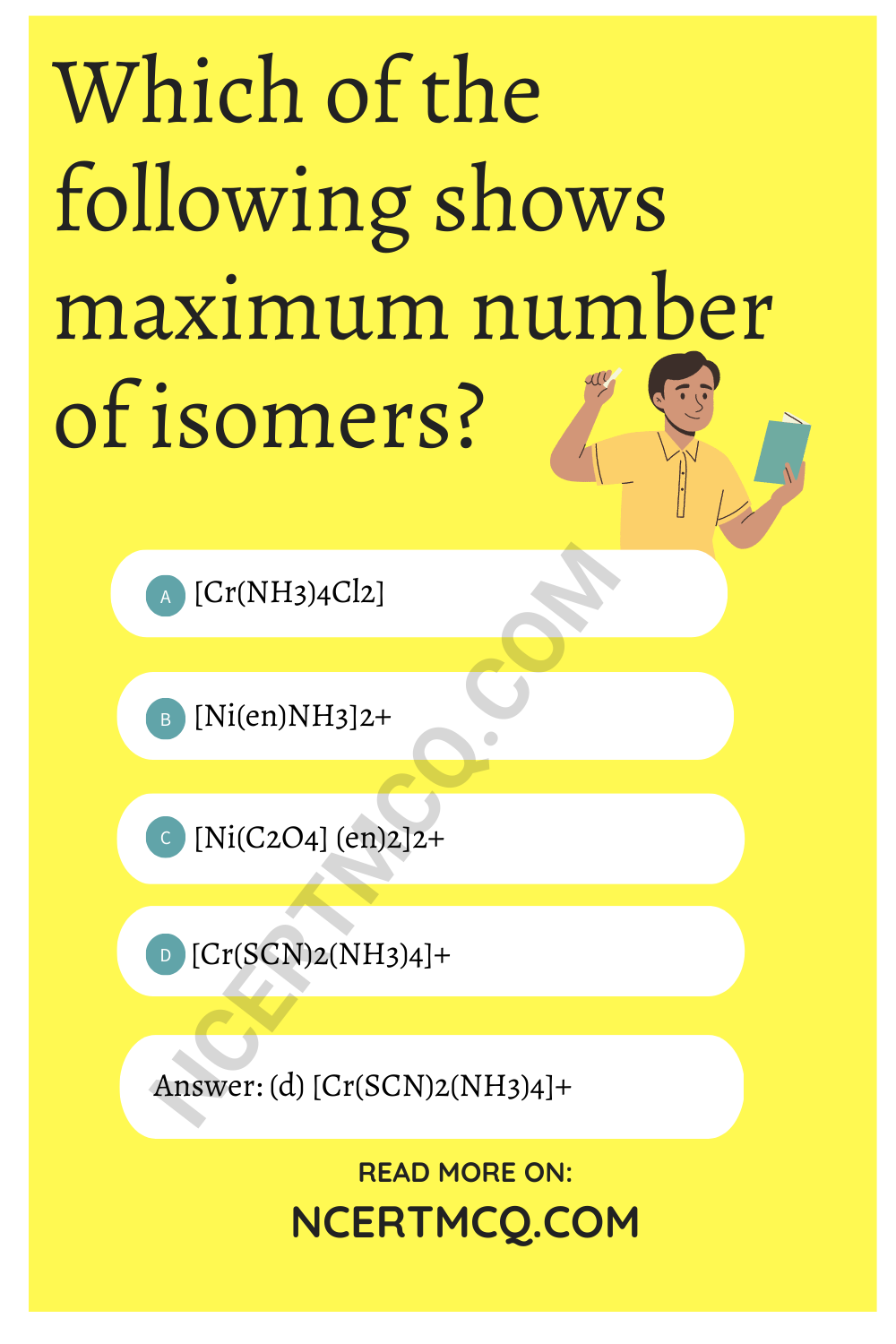Check the below NCERT MCQ Questions for Class 12 Chemistry Chapter 9 Coordination Compounds with Answers Pdf free download. MCQ Questions for Class 12 Chemistry with Answers were prepared based on the latest exam pattern. We have provided Coordination Compounds Class 12 Chemistry MCQs Questions with Answers to help students understand the concept very well.

## Coordination Compounds Class 12 MCQs Questions with Answers

Coordination Compounds MCQ With Answers Pdf Question 1.
K2[Fe(CN)6] is a/an
(a) double salt
(b) complex salt
(c) acid
(d) base

Coordination Compounds Class 12 MCQ Question 2.
Which of the following compounds has tetrahedral geometry?
(a) [Ni(CN)4]2-
(b) [Pd(CN)4]2-
(c [PdCl4]2-
(d) [NiCl4]2-

Coordination Is MCQ Class 12 Question 3.
The number of possible isomers for the complex
|Co(C2O4)2 (NH3)2|
(a) 1
(b) 2
(c) 3
(d) 4

Coordination Compounds MCQ Question 4.
The oxidation state of nickel in |(Ni(CO4)| is
(a) 1
(b) 4
(c) 2
(d) 3

MCQ On Coordination Compounds Question 5.
According to Werner’s theory of coordination compounds
(a) Primary valency is ion isable
(b) Secondary valency is ionisable
(c) Primary and secondary valencies are lonisable
(d) Neither primary nor secondary valency ¡s ionisable

Answer: (a) Primary valency is ion isable

MCQ Of Coordination Compounds Class 12 Question 6.
Ammonia acts as a very good ligand but ammonium ion does not form complexes because
(a) NH3 isa gas while NH$$_{4}^{+}$$ is in liquid form.
(b) NH3 undergoes sp3 hybridisatiort while NH$$_{4}^{+}$$ undergoes sp3 d hybndisation
(c) NH$$_{4}^{+}$$ ion does not have any lone pair of electrons
(d) NH$$_{4}^{+}$$ ion has one unpaired electron while NH3 has two unpaired electrons

Answer: (c) NH$$_{4}^{+}$$ ion does not have any lone pair of electrons

Coordination Compounds MCQ With Answers Question 7.
The ligand N(CH2CH2NH2) is
(a) bidentate
(b) tridentateCoordination Chemistry MCQ Question 8.
Which of the following is a tridentate ligand?
(a) EDTA4+
(b) (COO)$$_{2}^{2-}$$
(c) dien
(d) NO$$_{2}^{-}$$

Coordination Chemistry MCQ Questions And Answers Pdf Question 9.
Among the following which arc ambidentate ligands?
(i) SCN
(ii) NO$$_{3}^{-}$$
(iii) NO$$_{2}^{-}$$
(iv) C2O$$_{4}^{2-}$$
(a) (i) and (iii)
(b) (i) and (iv)
(c) (ii) and (iii)
(d) (ii) and (iv)

MCQs On Coordination Compounds Question 10.
Which of the following ligands form a chelate?
(a) Acetate
(b) Oxalate
(c) Cyanide
(d) Ammonia

Coordination Chemistry MCQ With Answers Question 11.
Which of the following is not a neutral ligand?
(a) H2O
(b) NH3
(c) ONO
(d) CO2

MCQ Questions On Coordination Compounds Question 12.
Which of the following ligands will not show chelation?
(a) EDTA
(b) DMG
(c) Ethene-1, 2-diamine
(d) SCN

MCQ On Coordination Chemistry Question 13.
The complex ion which has no d-electrons in the central metal atom is
(a) [MnO4]
(b) [Co(NH3)6]3-
(c) [Fe(CN)6]3-
(d) [Cr(H2O)6]3+

Coordination Compound MCQ Question 14.
The correct IUPAC name of the coordination compound K3|Fe(CN)5NO| is
(a) Potassium pentacyanonitrosylferrate (II)
(b) Potassium pentacyanonitroferrate (II)
(c) Potassium nitritopentacyanoferrate (IV)
(d) Potassium nitritepentacynanoiron (II)

Coordination Compounds MCQs Question 15.
The correct IUPAC name of the following compound is |Cr(NH3)5(NCS)||ZnCl4|
(a) Penta amine isothiocyanato chromium (III) tetrachlorozincate (II)
(b) Penta amine isothicocyanatezinc chloridechromate (III)
(c) Penta amine isothicoyanato chromate (II)
(d) isothiocyanatopenta amine chromium (II) zinc chloride (IV)

Answer: (a) Penta amine isothiocyanato chromium (III) tetrachlorozincate (II)

Question 16.
Correct formulae of tetraaminechloronitroplatinum (IV) sulphate can be written as
(a) [Pt(NH3)4 (ONO) Cl]SO4
(b) [Pt(NH3)4Cl2NO2]2
(c) [Pt(NH3)4 (NO2) Cl]SO4
(d) [PtCl(ONO)NH3(SO4)]

Question 17.
Which of the following isomers will give white precipitate with BaCl2 solution?
(a) [CO(NH3)5SO4]Br
(b) [CO(NH3)5Br]SO4
(c) [CO(NH3)4(SO4)2]Br
(d) [CO(NH3)4(SO4)]

Question 18.
|Pt(NH3)4| |CuCl4| and |Cu(NH3)4||PtCl4| are known as
(a) ionisation isomers.
(b) coordination isomers
(d) polymerisation isomers

Question 19.
The name of the linkage isomer of |C0(NH3)5NO2|Cl2 will be
(a) pentaammonotrocobalt (II) chloride
(b) pentaaminenitrochloridecobaltate (III)
(c) pentaamminenitritocobalt (III) chloride
(d) pentanitrosoamminechlorocobaltate(III)

Question 20.
Which of the following complex species is not expected to exhibit optical isomerism?
(a) [Co(en) (NH3)2Cl2]+
(b) [Co(en)3]3+
(c)[Co(en)2Cl2]
(d) [Co(NH3)3Cl3]

Question 21.
Which of the following compounds exhibits linkage isomerism?
(a) [Co(en)3]Cl3
(b) [Co(NH3)6] [Cr(en)3]
(c) [Co(en)2(NO2)CI]Br
(d) [Co(NH3)5CI]Br2

Question 22.
Which of the following will not show geometrical isomerism?
(a) [Cr(NH3)4Cl2]Cl
(b) [Co(en)2Cl2]Cl
(c) [(Co(NH3)5NO2]Cl2
(d) [Pt(NH3)2Cl2]

Question 23.
Which of the following shows maximum number of isomers?
(a) [Cr(NH3)4Cl2]
(b) [Ni(en)NH3]2+
(c) [Ni(C2O4] (en)2]2+
(d) [Cr(SCN)2(NH3)4]+Question 24.
For the square planar complex |Mabcd| where M is the central atom and a, b. c, d are monodentate ligands, the number of possible geometrical isomers are
(a) 1
(b) 2
(c) 3
(d) 4

Question 25.
The hybridisation involved in [Co(C2O4)3]3- is
(a) sp3d2
(b) sp3d3
(c) dsp3
(d) d2sp3